# Special relativity and time dilation

DanPease
I was just looking for a basic explanation of special relativity in relation to an object with mass accelerating towards the speed of light. And with reference too time dilation can v and c be referred too as a percentage or decimal depending on the units in the equation used??

Many Thanks

## Answers and Replies

Mentor
Welcome to PF!

1. Thread moved to the Relativity section.
2. Could you please be more specific about what you want explained? What resources have you used so far?
3. Yes, in some contexts, C can just be 1 (1C) and velocity can be a fraction of it.

DanPease
Well I wanted too know why an object with mass can't travel at the speed of light with reference to SR.

Gold Member
2021 Award
Well I wanted too know why an object with mass can't travel at the speed of light with reference to SR.

Because it would take infinite energy to get it go c

Noyhcat
Well I wanted too know why an object with mass can't travel at the speed of light with reference to SR.

Because it would take infinite energy to get it go c

Right, because as I understand it, it's because when you apply the Lorentz factor ("with reference to SR") to things like $F=ma$, you actually get $F = \gamma ma$. Which is:

$F= \frac{ma}{\sqrt{1-\frac{v^2}{c^2}}}$.

As you can see, when velocity increases, the amount of force required to accelerate it approaches infinity.

dauto
Right, because as I understand it, it's because when you apply the Lorentz factor ("with reference to SR") to things like $F=ma$, you actually get $F = \gamma ma$. Which is:

$F= \frac{ma}{\sqrt{1-\frac{v^2}{c^2}}}$.

As you can see, when velocity increases, the amount of force required to accelerate it approaches infinity.

That's not right. You only get $F = \gamma ma$ if the acceleration is perpendicular to the velocity. If they are parallel to each other you'll get $F = \gamma^3 ma$, and if it is neither parallel nor perpendicular, you get the more general $\vec F = \gamma m[\vec a + \frac{\gamma^2}{c^2}(\vec a \cdot \vec u)\vec u]$ which means the force and the acceleration won't even be parallel to each other.

DanPease
Sweet guys thanks for the help. Would anyone be kind enough too explain length contraction??

dauto
Yes, moving objects will have their length contracted by a $\gamma$ factor. Of course from an object's own point of view it is never moving so there is no contraction. But other observers will see the object contracted according with how fast the object is moving from their point of view.

DanPease
Brilliant, cheers buddy.

Noyhcat
That's not right. You only get $F = \gamma ma$ if the acceleration is perpendicular to the velocity. If they are parallel to each other you'll get $F = \gamma^3 ma$, and if it is neither parallel nor perpendicular, you get the more general $\vec F = \gamma m[\vec a + \frac{\gamma^2}{c^2}(\vec a \cdot \vec u)\vec u]$ which means the force and the acceleration won't even be parallel to each other.

Figured I'd start simple for clarity and go with the $\perp$.DanPease
Ok guys, why does time dilation happen?? What is it about the speed of light that warps time???

Ok guys, why does time dilation happen?? What is it about the speed of light that warps time???
Because the speed of light is the same for all observers. Time dilation results from this fact. Why is the speed of light constant for all observers? Who knows...ask the Flying Spaghetti Monster.

Gold Member
2021 Award
Ok guys, why does time dilation happen?? What is it about the speed of light that warps time???

It's a bit of a mistake to think of it as "warping time". What happens is that from one inertial frame of reference X, the time for a fast-moving object (fast-moving relative to X) appears to be slower than it appears for the clock that is traveling along with the fast-moving object.

The hitch is that the clock that is moving along with the "fast-moving" object is NOT moving relative to itself and so it ticks at the same one second per second that the clock in X does, and it does not experience any time dilation.

So time dilation is an artifact of remote observation, not a physical reality.

BUT ... to further complicate things, if the two clocks are brought back together (which requires the "fast moving" one to accelerate) they WILL show different times because they have traveled along different world-lines to arrive at the even which is their reunion.

This is discussed at length with the "twin paradox".

So time dilation is an artifact of remote observation, not a physical reality.
It has nothing to do with remote observation. A moving object will age slower even when it is very close.

Gold Member
2021 Award
It has nothing to do with remote observation. A moving object will age slower even when it is very close.

But only in the reference frame of the person making the measurement, which is why I say it IS an artifact of remote observation.

For example, if you have X1 taken as a base-line frame of reference and you have X2 moving at some speed away from X1 and you have X3 moving at that same speed away from X2 (and thus at [almost] double the speed relative to X1) then the time passage in X3 will be seen as one thing in X3, a slower amount in X2 and a still slower amount in X1.

Which of these is right? They are all correct, but only in their own reference frames. They are not absolute and the "slower" measurements of X1 and X2 are just as correct as the measurement in X3. Thus, I say that the "slowness" of X3 is an artifact of remote observation and have no effect on the time measurements taken in X3.

Last edited:
dauto
Couple quibbles: X3 will not be seen at double of the speed from X1 point of view. Also, while what you said is essentially correct, the word artifact is a poor choice because it is often used to describe effects that aren't actually real. Time dilation is real.

yuiop
Couple quibbles: X3 will not be seen at double of the speed from X1 point of view. Also, while what you said is essentially correct, the word artifact is a poor choice because it is often used to describe effects that aren't actually real. Time dilation is real.

It is just unfortunate that we do not have a rigorous definition of real in this context. If by real we mean a coordinate independent measurement that all observers agree on, then the relative time dilation between two strictly inertial reference frames is not real.

Gold Member
2021 Award
Couple quibbles: X3 will not be seen at double of the speed from X1 point of view.

Yeah, I was over-simplifying.

Also, while what you said is essentially correct, the word artifact is a poor choice because it is often used to describe effects that aren't actually real. Time dilation is real.

Hm ... I'll have to think about that one.

dauto
It is just unfortunate that we do not have a rigorous definition of real in this context. If by real we mean a coordinate independent measurement that all observers agree on, then the relative time dilation between two strictly inertial reference frames is not real.

Good thing that's not what's meant by real uh?DanPease
So with reference too time dilation is it only the clocks that are affected not the physical effects of time?

yuiop
So with reference too time dilation is it only the clocks that are affected not the physical effects of time?
All physical effects of time are affected in the same way as clocks. For example lets say you light a small candle and it takes one hour to burn down. Now you are accelerated to 0.8c relative to Joe and you light another similar candle. It still takes one hour to burn down according to your clock so everything seems normal to you, but Joe will say it took one hour and 40 minutes to burn down by his clock. The candle is of course a primitive form of clock and any local physical dynamic process such as your biological ageing is also effectively a clock.

Last edited:
Gold Member
2021 Award
So with reference too time dilation is it only the clocks that are affected not the physical effects of time?

Did you not understand post #15 or did you just not believe it?

Noyhcat
I tend to think of it this way: acceleration doesn't affect our universe but rather reveals it.

That c in a vacuum is the same in all reference frames doesn't "cause" anything to happen, but rather reveals to us why things happen, or how maybe.

Acceleration and light don't distort time. They simply reveal its distorted nature.

Gold Member
Well I wanted too know why an object with mass can't travel at the speed of light with reference to SR.

Sweet guys thanks for the help. Would anyone be kind enough too explain length contraction??

Ok guys, why does time dilation happen?? What is it about the speed of light that warps time???

The best way I know to answer all your questions is for you to learn about the Lorentz Transformation process and to draw spacetime diagrams. I'll start with a simple situation where an observer, shown in blue, is measuring the round-trip speed of light by sending a pulse of light towards a mirror shown in red that is mounted 3 feet away. He has a clock that measures the time the light is emitted and the time the reflection gets back to himself. Since the speed of light is one foot per nanosecond, his clock advances by 6 nanoseconds during the time the light propagates 3 feet to the mirror (shown as the thin blue line) and 3 feet back (shown as the thin red line). I've shown the observer's time in blue. The time for the mirror is shown in red. The dots mark off 1-nanosecond increments of time for the observer and for the mirror. Note that these two times are not synchronized: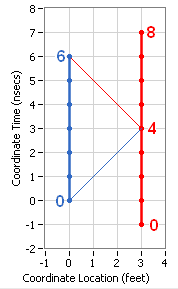Now I'm going to transform the coordinates of all the events (the dots) to a new frame moving at 0.6c (or 0.6 feet per nsec):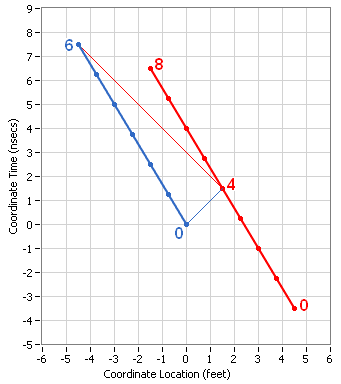The Time Dilation factor at 0.6c is 1.25. Note that the dots are stretched out so that 4 nsec for each clock takes 4 times 1.25 or 5 nsec of Coordinate Time.

Also note that the spacing between the observer and the mirror has been reduced from 3 feet to 3/1.25=2.4 feet. To see this, look at the Coordinate Time of 0 and you will see that the observer is at 0 feet while the mirror is at 2.4 feet.

And finally note that the pulse of light and its reflection continue to propagate at 1 foot per nsec and is emitted by the observer, reflected at the mirror, and received by the observer with the same times as in the original frame.

Now we want to add in the configuration from the first diagram as an extension to this diagram: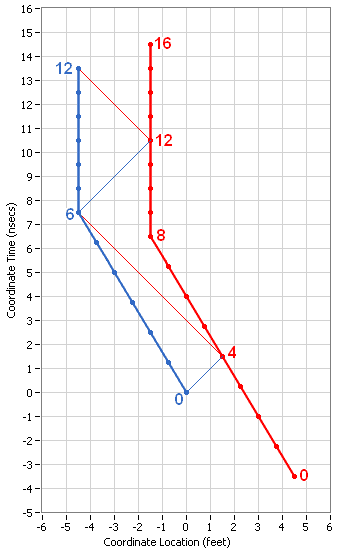This represents an acceleration of the observer and an earlier acceleration of the mirror such that they are both at rest in the new frame. The observer makes the same measurement of the round-trip speed of light as he did before accelerating, just with his clock advanced by 6 nsec (and the time on the mirror advanced by 8 nsec). Note that the two times are even more out of sync than they were during the first segment.

We can repeat the process again: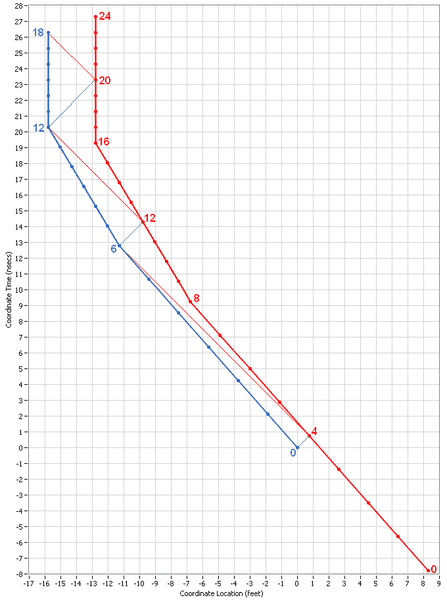Note that the observer again has the same experience as before, just with his clock advanced by 6 more nsecs and the time for the mirror advanced by 8 nsec and the two times getting more out of synch. Also note that the previous segment has the same Time Dilation and Length Contraction as the original segment did in the previous frame and the original segment in this frame has more Time Dilation and more Length Contraction than it did in the previous frame.

Now I'm going to repeat the process one more time but I'm only going to show the last part of the scenario in the same scale as the previous one: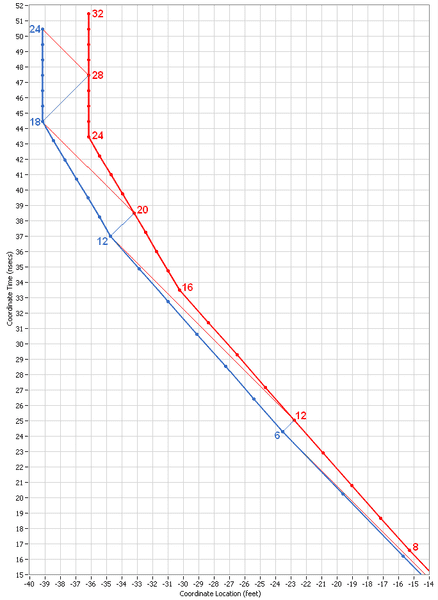Aside from the differences in the coordinates and the advancing of the times, you can see the exact same image of the previous diagram in this one and we could repeat the process as many times as we wanted with the same result. In other words, no matter how much the observer accelerates, he is no closer to the speed of light than when he started.

Here is one more diagram showing the entire scenario but drawn at one-half the scale so that it will fit on one page: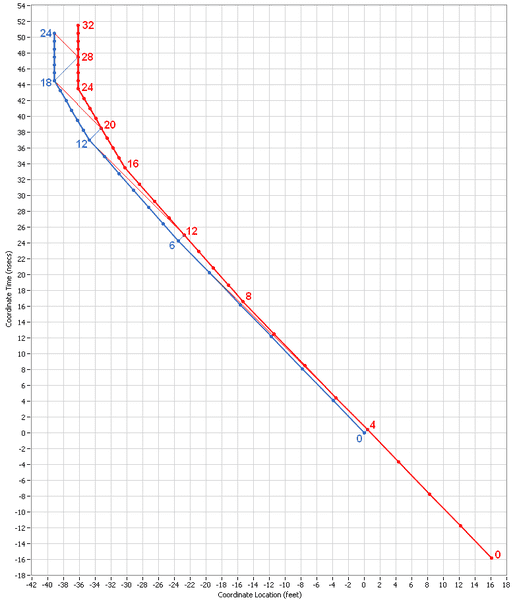Any questions?

#### Attachments

Naty1
Nobody arrived at the fact the the speed of light is viewed as, is, a constant from all inertial frames, from pure logic...nor from first principles...it was a mystery. People struggled to understand if it was true...and how to explain it. Even once you accept the idea, which seemed somewhat crazy at the time, that the speed of light is constant, it still took and "Einstein' to put together a theory.

The history is outlined ..and the formulas discussed..... here:

In this universe, 'c' is constant, space is not, time is not....even though they seem to be 'fixed' to our everyday senses.

Why can't any mass reach 'c'??: because no matter how fast one goes, light still whizzes by locally at 'c'....you just can't catch up. Everybody measures 'c' locally!! It IS "crazy".

It turns out the Lorentz Transformation [formula], which Einstein incorporated in Special Relativity, describes what we observe; from there you solve for length or for time change as follows:

From the above Wikipedia link, Length contraction is the first formula here:

Basis in relativity

http://en.wikipedia.org/wiki/Fitzgerald_contraction#Basis_in_relativity
for L = ..........

and from the same Wikipedia link, time dilation is the first formula here:

Time Dilation

http://en.wikipedia.org/wiki/Fitzgerald_contraction#Time_dilation

T = .............